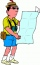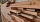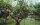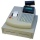# Goniomentry and trigonometry - problems

1. TouristA tourist walked an average speed of 3.5 km/h route in 6 hours. Calculate how many hours he would have passed at an average speed of 5.5km/h.
2. The workThe work was to be done by 150 workers. At the beginning of their work, their number reduced by 40, which increased the time of work by 5 and 1/3 of the schedule. How long did work take?
3. Isosceles triangle 8If the rate of the sides an isosceles triangle is 7:6:7, find the base angle correct to the nearest degree.
4. Four workersFour workers will lay the floor of the gym in six hours. After an hour of working together, one of the workers left to the doctor. How long before the remaining three workers finish their work?
5. LumberjacksFifteen lumberjacks would take 12 days to destroy the broken trees. How many days would this work require if 5 workers added to it after 4 days?
6. Ten cashiersTen cashiers are open at Tesco. Customers wait an average of 15 minutes. How many other cashiers have to open to reduce the waiting time by 4 minutes?
7. Trapezoid MOThe rectangular trapezoid ABCD with right angle at point B, |AC| = 12, |CD| = 8, diagonals are perpendicular to each other. Calculate the perimeter and area of ​​the trapezoid.
8. Trigonometric functionsIn right triangle is: ? Determine the value of s and c: ? ?
9. Triangle SASCalculate area and perimeter of the triangle, if the two sides are 51 cm and 110 cm long and angle them clamped is 130°.
10. RiverFrom the observatory 14 m high and 32 m from the river bank, river width appears in the visual angle φ = 20°. Calculate width of the river.
11. Regular 5-gonCalculate area of the regular pentagon with side 7 cm.
12. ObserverThe observer sees straight fence 100 m long in 30° view angle. From one end of the fence is 153 m. How far is it from the another end of the fence?
13. ForcesIn point O acts three orthogonal forces: F1 = 20 N, F2 = 7 N and F3 = 19 N. Determine the resultant of F and the angles between F and forces F1, F2 and F3.
14. TriangleTriangle KLM is given by plane coordinates of vertices: K[-12, -11] L[-15, -18] M[-13, -12]. Calculate its area and itsinterior angles.
15. RightDetermine angles of the right triangle with the hypotenuse c and legs a, b, if: ?
16. IS triangleCalculate interior angles of the isosceles triangle with base 38 cm and legs 26 cm long.
17. Cuboid diagonalCalculate the volume and surface area of the cuboid ABCDEFGH, which sides abc has dimensions in the ratio of 9:3:8 and if you know that the wall diagonal AC is 86 cm and angle between AC and the body diagonal AG is 25 degrees.
18. ConeCalculate volume and surface area of ​​the cone with diameter of the base d = 15 cm and side of cone with the base has angle 52°.
19. Slope of trackCalculate the average slope (in promiles and even in degrees) of the rail tracks between Prievidza (309 m AMSL) and Topoľčany (174 m AMSL), if the track is 44 km long.
20. PentagonCalculate the area of regular pentagon, which diagonal is u=17.

Do you have an interesting mathematical problem that you can't solve it? Enter it, and we can try to solve it.

To this e-mail address, we will reply solution; solved examples are also published here. Please enter e-mail correctly and check whether you don't have a full mailbox.

Most natural application of trigonometry and trigonometric functions is a calculation of the triangles. Common and less common calculations of different types of triangles offers our triangle calculator. Word trigonometry comes from Greek and literally means triangle calculation.